﻿ 高速凸轮纯滚动条件下的润滑过程分析

# 高速凸轮纯滚动条件下的润滑过程分析Numerical Analysis of Lubrication Process in High-Speed Cam-Roller Contact

Abstract: In this paper, the multigrid method and multilevel multi-integration technique are used to obtain the numerical solution of thermal EHL in smooth line contact for a cam-roller mechanism. The pressure, film thickness and temperature rise profiles at a number of angular positions of cam are presented. The thermal rise is negligibly small in the cam-roller contact. The characteristics of the oil film are given. Then, a roller with larger radius is studied to show the variations of oil charac-teristics.

1. 引言

2. 数学模型

$\frac{\partial }{\partial x}\left[{\left(\frac{\rho }{\eta }\right)}_{e}{h}^{3}\frac{\partial p}{\partial x}\right]=6{u}_{a}\frac{\partial }{\partial x}\left({\rho }_{a}^{*}h\right)+6{u}_{b}\frac{\partial }{\partial x}\left({\rho }_{b}^{*}h\right)+12\frac{\partial }{\partial t}\left({\rho }_{e}h\right)$ (1)

${\rho }_{a}^{*}=2\left({\rho }_{e}-{{\rho }^{\prime }}_{e}{\eta }_{e}\right),{\left(\rho /\eta \right)}_{e}=12\left({\eta }_{e}{{\rho }^{\prime }}_{e}/{{\eta }^{\prime }}_{e}-{{\rho }^{″}}_{e}\right)$

${\rho }_{b}^{*}=2{{\rho }^{\prime }}_{e}\eta ,{\rho }_{e}=\left(1/h\right){\int }_{0}^{h}\rho \text{d}z$

${{\rho }^{\prime }}_{e}=\left(1/{h}^{2}\right){\int }_{0}^{h}\rho {\int }_{0}^{z}\text{1}/\eta \text{d}{z}^{\prime }\text{d}z,{{\rho }^{″}}_{e}=\left(1/{h}^{3}\right){\int }_{0}^{h}\rho {\int }_{0}^{z}{z}^{\prime }/\eta \text{d}{z}^{\prime }\text{d}z$

${\eta }_{e}=h/{\int }_{0}^{h}1/\eta \text{d}z,{{\eta }^{\prime }}_{e}={h}^{2}/{\int }_{0}^{h}z/\eta \text{d}z$

Reynolds方程边界条件

$\left\{\begin{array}{l}p\left({x}_{\text{in}},t\right)=p\left({x}_{\text{out}},t\right)=0\\ p\left(x,t\right)\ge 0\text{ }\left({x}_{\text{in}} (2)

$h\left(x,t\right)={h}_{00}\left(t\right)+\frac{{x}^{2}}{2R}+\frac{2}{\text{π}{E}^{\prime }}\int \int p\left({x}^{\prime },t\right)\mathrm{ln}\left(x-{x}^{\prime }\right)\text{d}{x}^{\prime }$ (3)

$\iint p\left(x,t\right)\text{d}x={w}_{0}+{k}_{s}\ast s/L+\left({m}_{s}/3+{m}_{t}+{m}_{v}\right)\ast \frac{{\text{d}}^{2}s}{\text{d}{t}^{2}}*\frac{1}{L}$ (4)

$\rho ={\rho }_{0}\left(1+\frac{{C}_{1}p}{1+{C}_{2}p}-{C}_{3}\left(t-{t}_{0}\right)\right)$ (5)

$\eta ={\eta }_{0}\mathrm{exp}\left\{{A}_{1}\left[-1+{\left(1+{A}_{2}p\right)}^{{Z}_{0}}{\left({A}_{3}t-{A}_{4}\right)}^{-{S}_{0}}\right]\right\}$ (6)

$c\left(\rho u\frac{\partial T}{\partial x}+\rho \frac{\partial T}{\partial t}-w\frac{\partial T}{\partial z}\right)-k\frac{{\partial }^{2}T}{\partial {z}^{2}}=-\frac{T}{\rho }\frac{\partial \rho }{\partial T}\left(u\frac{\partial p}{\partial x}+\frac{\partial p}{\partial t}\right)+\tau \frac{\partial u}{\partial z}$ (7)

$\left\{\begin{array}{l}T\left({x}_{\text{in}},z,t\right)={T}_{0}\text{ }\left(u\left({x}_{in},z,t\right)\ge 0\right)\\ T\left({x}_{\text{out}},z,t\right)={T}_{0}\text{ }\left(u\left({x}_{\text{out}},z,t\right)\le 0\right)\end{array}$ (8)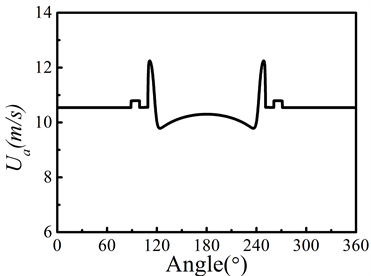(a)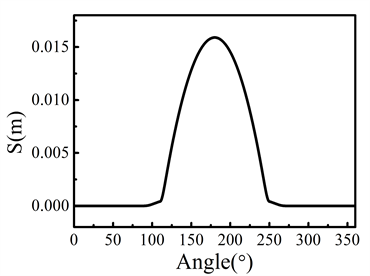(b)

Figure 1. Kinematic conditions of the cam-tapper pair over one period, R1 = 10 mm, ω = 65 π rad/s

$\left\{\begin{array}{l}{c}_{a}{\rho }_{a}\left(\frac{\partial T}{\partial t}+{u}_{a}\frac{\partial T}{\partial x}\right)={k}_{a}\frac{{\partial }^{2}T}{\partial {z}_{a}^{2}}\\ {c}_{b}{\rho }_{b}\left(\frac{\partial T}{\partial t}+{u}_{b}\frac{\partial T}{\partial x}\right)={k}_{b}\frac{{\partial }^{2}T}{\partial {z}_{b}^{2}}\end{array}$ (9)

$\left\{\begin{array}{l}k{\frac{\partial T}{\partial z}|}_{z=0}={k}_{a}{\frac{\partial T}{\partial {z}_{a}}|}_{{z}_{a}=0}\\ k{\frac{\partial T}{\partial z}|}_{z=h}={k}_{b}{\frac{\partial T}{\partial {z}_{b}}|}_{{z}_{b}=0}\end{array}$ (10)

2. 数值方法

$P=p/{p}_{\text{H}}$ , $h=h{R}_{0}/{b}^{2}$ , $X=x/b$ , $Z=z/h$ , ${Z}_{a,b}={z}_{a,b}/b$

$\stackrel{¯}{T}=T/{T}_{0}$ , ${\stackrel{¯}{T}}_{a,b}={\stackrel{¯}{T}}_{a,b}/{T}_{0}$ , $\stackrel{¯}{\tau }=\tau /{p}_{\text{H}}$

$U=u/{u}_{0}$ , ${U}_{0}={u}_{0}{\eta }_{0}/{E}^{\prime }{R}_{0}$ , ${U}_{a,b}={u}_{a,b}/{u}_{0}$ , $\stackrel{¯}{\eta }=\eta /{\eta }_{0}$

$\stackrel{¯}{\rho }=\rho /{\rho }_{0}$ , $W={w}_{0}/\left({E}^{\prime }R\right)$ , $G=\alpha {E}^{\prime }$

3. 结果和讨论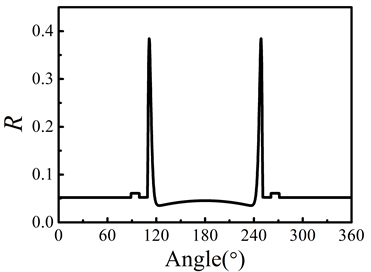(a)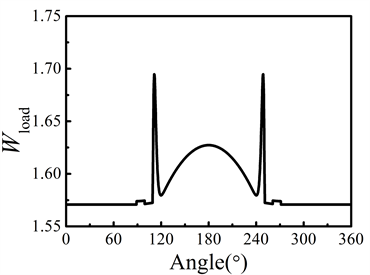(b)

Figure 2. Kinematic conditions of the cam-tapper pair over one period, R1 = 10 mm, ω = 65 π rad/s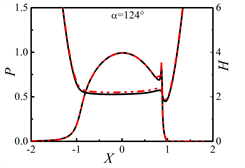(a) α = 88˚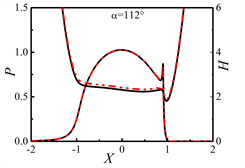(b) α = 112˚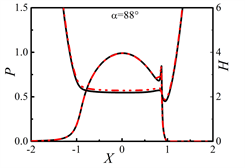(c) α = 124˚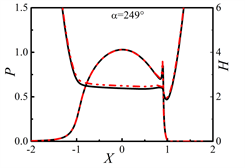(d) α = 180˚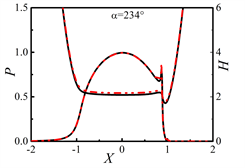(e) α = 234˚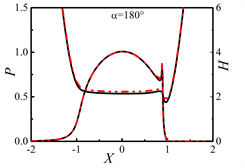(f) α = 249˚

Figure 3. Variations of film thickness profiles over one period, R0 = 10 mm, ω = 65π rad/sFigure 4. Variations of thermal oil characteristics over one period, R0 = 10 mm, ω = 65 π rad/s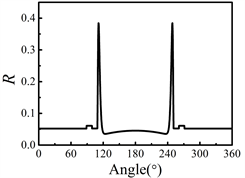Figure 5. Equivalent radius of curvature over one period, R1 = 15 mm, ω = 65 π rad/s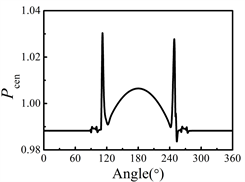Figure 6. Variations of thermal oil characteristics over one period, R0 = 15 mm, ω = 60 π rad/s

4. 结论

a、凸轮-滚子挺杆在纯滚动运动情况下，油膜的变化主要受载荷的影响。在凸轮桃尖的两侧转角α = 119˚时，油膜厚度和中心压力均会产生较大的波动，并且在该点附近油膜厚度达到最低值。

b、当适当增加滚子的半径，油膜的厚度有了明显的提升，油膜的温升也随之变大且波动变得明显。但是由于是纯滚动，产生热量对油膜影响不大。总而言之，适当增加滚子的半径有助于凸轮-滚子挺柱的润滑。

 Khurram, M. and Mufti, R.A. (2015) Experimental Measurement of Roller Slip in End-Pivoted Roller Follower Valve Train. Proceedings of the Institution of Mechanical Engineers, Part J: Journal of Engineering Tribology, 229, 1047-1055.
https://doi.org/10.1177/1350650115572198

 Alakhramsing, S.S., de Rooij, M.B., Schipper, D.J. and van Drogen, M. (2017) A Full Numerical Solution to the Coupled Cam-Roller and Roller-Pin Contact in Heavily Loaded Cam-Roller Follower Mechanisms. Proceedings of the Institution of Mechanical Engineers, Part J: Journal of Engineering Tribology.
https://doi.org/10.1177/1350650117746899

 Alakhramsing, S.S., de Rooij, M.B., Schipper, D.J. and van Drogen, M. (2018) Lubrication and Frictional Analysis of Cam-Roller Follower Mechanisms. Proceedings of the In-stitution of Mechanical Engineers, Part J: Journal of Engineering Tribology, 232, 347-363.

 Alakhramsing, S.S., de Rooij, M.B., Schipper, D.J. and van Drogen, M. (2018) The Influence of Stick-Slip Transitions in Mixed-Friction Predictions of Heavily Loaded Cam-Roller Contacts. Proceedings of the Institution of Mechanical Engineers, Part J: Journal of Engineering Tribology.
https://doi.org/10.1177/1350650118789515

 Kushwaha, M. and Rahnejat, H. (2002) Transient Elastohydrodynamic Lubrication of Finite Line Conjunction of Cam to Follower Concentrated Contact. Journal of Physics D: Applied Physics, 35, 2872.
https://doi.org/10.1088/0022-3727/35/21/327

 Yang, P. and Wen, S. (1990) A Generalized Reynolds Equation for Non-Newtonian Thermal Elastohydrodynamic Lubrication. Journal of Tribology, 112, 631-636.
https://doi.org/10.1115/1.2920308

Top